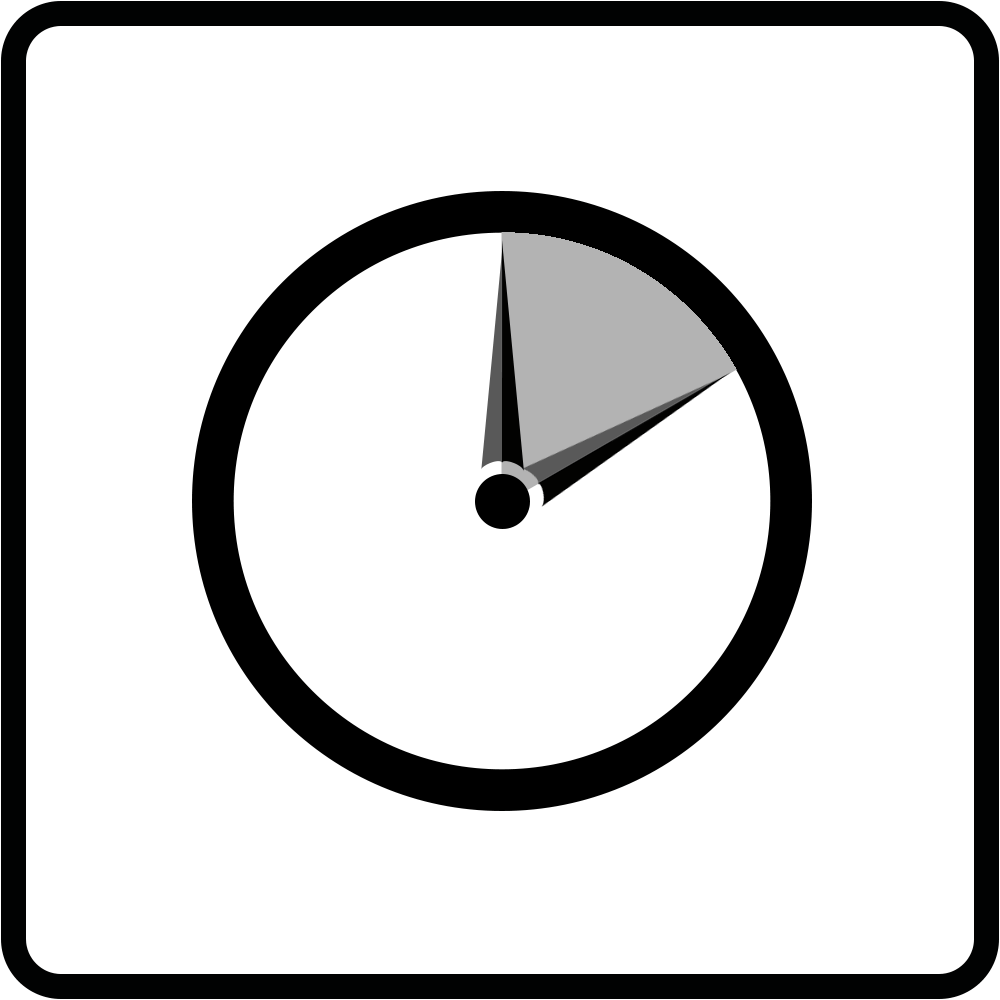Target Zer0 is a straightforward dice rolling competition. Players roll a number of dice and use a pre-determined set of mathematical operators to construct an equation that will produce a result as close to zero as possible.

Educational: Players are required to practice their mathematical skills by utilizing the various mathematical operators, order of operations, and sub-expressions to design the best equations they can.Ages 7+2+ players5-15 min.